# scikit-learn之kmeans应用及问题

### scikit-learn之kmeans应用及问题

• sklearn中KMeans的参数及应用
• 聚类效果的评估指标——轮廓系数和Calinski-Harabaz Index
• 如何选择最佳的簇的个数——“肘部”法
• sklearn中KMeans大数据量下如何优化
• sklearn中MiniBatchKmeans的参数及应用

sklearn中KMeans的主要参数如下：

n_clusters 这个就是k值，设置的簇的个数
init 簇心初始化的方法，有k-means++初始化方法，随机（random）初始化和自己指定样本（an ndarray），默认为k-means++，一般用默认的就可以，k-means++初始化效果也比较好
n_init 用不同的初始化簇心运行算法的次数，默认值为10，即运行10次，每次初始化的簇心都不相同，最后算法会选一个最好的结果。
max_iter 最大迭代次数，这个参数主要是为了防止k-means不收敛，到达最大迭代次数后算法就终止
tol 容忍的最小误差，当误差小于tol时算法就会终止
precompute_distances 这个参数是权衡时间和空间。有三个值：true，false，auto。true的话会把整个矩阵放到内存，占用内存大点，但是时间开销小。false反之。auto就是当n_samples * n_clusters > 12 million时为false，小于时为true。默认为auto，一般任务默认就可以。
verbose 详细模式，就是设置为true的话会打出运行时的细节过程，建议设置为true，因为这样能更好的知道程序运行到什么程度了。
n_jobs 多线程的，默认为1，如果是-1则表示用该机的所有线程。这个并行是和上面 n_init 这个参数对应的，也就是说如果这边设置了并行，上面 n_init 设置的初始化簇心运行算法的次数，会被并行的执行
algorithm 有“auto”, “full” or “elkan”三种选择。“full"是传统的K-Means算法， “elkan”是elkan K-Means算法。默认的"auto"则会根据数据值是否是稀疏的，来决定如何选择"full"和“elkan”。一般数据是稠密的，那么就是 “elkan”，否则就是"full”。一般可以直接用默认的"auto"

from sklearn.datasets.samples_generator import make_blobs
import matplotlib.pyplot as plt

X, y = make_blobs(n_samples=1000, centers=[[-9,-8],[-10,6],[-10,0],[-5,-1],[0,7],[-2,3],[0,-8],[10,-5],[5, 0],[8,7]], n_features=2, random_state=1)
print(X.shape)
plt.scatter(X[:,0], X[:,1],c = y, marker = 'o', s = 25, cmap = plt.cm.get_cmap("jet",10))
plt.show()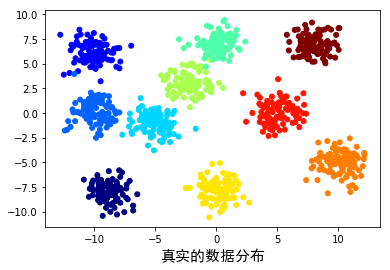from sklearn.cluster import KMeans
#k=3
y_pred_3 = KMeans(n_clusters=3, verbose = 1, n_jobs = -1, random_state=1).fit_predict(X)
plt.scatter(X[:,0], X[:,1],c = y_pred_3, marker = 'o', cmap = plt.cm.get_cmap("jet",3))
plt.show()

#k=6
y_pred_6 = KMeans(n_clusters=6, verbose = 0, n_jobs = -1, random_state=1).fit_predict(X)
plt.scatter(X[:,0], X[:,1],c = y_pred_6, marker = 'o', cmap = plt.cm.get_cmap("jet",6))
plt.show()

#k=10
y_pred_10 = KMeans(n_clusters = 10, verbose = 0, n_jobs = -1, random_state=1).fit_predict(X)
plt.scatter(X[:,0], X[:,1],c = y_pred_10, marker = 'o', cmap = plt.cm.get_cmap("jet",10))
plt.show()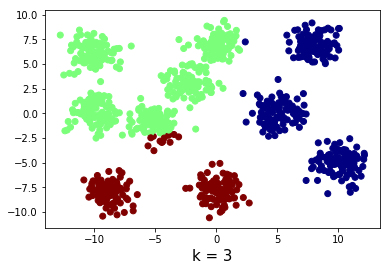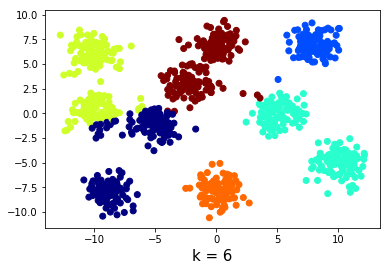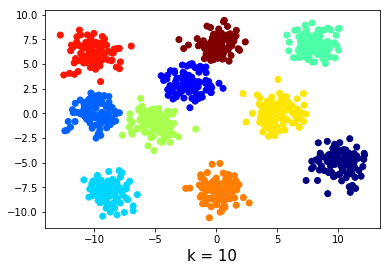对于没有真实label的数据，评估聚类效果常用两种指标：Silhouette Coefficient（轮廓系数）和Calinski-Harabasz Index，在sklearn中分别对应的实现为：silhouette_score和calinski_harabasz_score。

silhouette_score的公式为：
$sc = \frac{b-a}{max(a,b)}$

calinski_harabaz_scores的公式为：
$s(k) = \frac{\mathrm{Tr}(B_k)}{\mathrm{Tr}(W_k)} \times \frac{N - k}{k - 1}$
$W_k = \sum_{q=1}^k \sum_{x \in C_q} (x - c_q) (x - c_q)^T \\ B_k = \sum_q n_q (c_q - c) (c_q - c)^T$

silhouette_score和calinski_harabaz_scores的区别：

silhouette_score的结果更加准确，但是其计算所需时间也长，甚至可能出现内存爆掉的可能，因此如果数据量很大，不建议用这个评估指标，建议用calinski_harabasz_score。calinski_harabasz_score计算的结果比较粗糙，但是其时间开销非常小，计算的很快。

silhouette_scores

import time
from sklearn.metrics import silhouette_score,calinski_harabaz_score
clusters = [2, 3, 4, 5, 6, 7, 8, 9, 10, 11, 12, 13, 14]
silhouette_scores = []
#轮廓系数silhouette_scores
print('start time: ',time.strftime('%Y-%m-%d %H:%M:%S',time.localtime(time.time())))
for k in clusters:
y_pred = KMeans(n_clusters = k, verbose = 0, n_jobs = -1, random_state=1).fit_predict(X)
score = silhouette_score(X, y_pred)
silhouette_scores.append(score)
print('finish time: ',time.strftime('%Y-%m-%d %H:%M:%S',time.localtime(time.time())))
plt.plot(clusters, silhouette_scores, '*-')
plt.xlabel('k')
plt.ylabel('silhouette_score')
plt.show()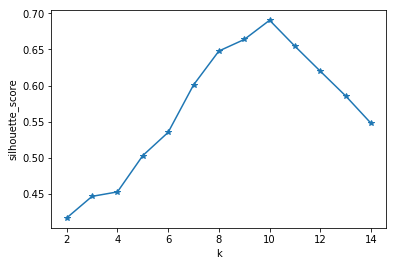calinski_harabaz_scores

calinski_harabaz_scores = []
#calinski_harabasz_scores
print('start time: ',time.strftime('%Y-%m-%d %H:%M:%S',time.localtime(time.time())))
for k in clusters:
y_pred = KMeans(n_clusters = k, verbose = 0, n_jobs = -1, random_state = 1).fit_predict(X)
score = calinski_harabaz_score(X, y_pred)
calinski_harabaz_scores.append(score)
print('finish time: ',time.strftime('%Y-%m-%d %H:%M:%S',time.localtime(time.time())))
plt.plot(clusters, calinski_harabaz_scores, '*-')
plt.xlabel('k')
plt.ylabel('calinski_harabaz')
plt.show()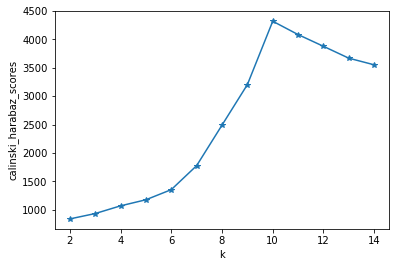“肘部”观察法可以粗略的预估比较合理的簇的个数（ps.只是粗略的预估相对合理），因为k-means最终收敛的时候，所有数据点到其所属的类簇距离的平方和趋于稳定，所以我们可以通过观察这个数值随着k的走势来找出相对最好的类簇数量。理想条件下，这个折线在不断下降并且趋于平缓的过程中会有斜率的拐点，同时意味着从这个拐点对应的k值开始，簇中心的增加不会过于破坏数据聚类的结构。但其实这个方法不适用于大数量样本，因为要把不同k值跑一遍。

import numpy as np
from scipy.spatial.distance import cdist
mean_distances = []
print('start time: ',time.strftime('%Y-%m-%d %H:%M:%S',time.localtime(time.time())))
for k in clusters:
kmeans = KMeans(n_clusters = k, verbose = 0, n_jobs = -1, random_state = 1).fit(X)
mean_distances.append(sum(np.min(cdist(X, kmeans.cluster_centers_, 'euclidean'), axis=1))/X.shape)
print('finish time: ',time.strftime('%Y-%m-%d %H:%M:%S',time.localtime(time.time())))
plt.plot(clusters, mean_distances, '*-')
plt.xlabel('k')
plt.ylabel('mean_distances')
plt.show()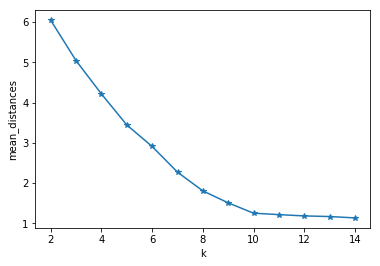NOTEsklearn的kmeans在小数量下跑的很 happy，完全没有任何问题。但是当遇到大数量情况下（比如我开头提到的，10G左右的数据集，370w*200），完全扛不住，基本上都是内存爆掉。这时候的解决办法有两种，第一种就是从kmeans的参数上做文章进行优化，但这种方法治标不治本。因此强烈建议使用第二种方法，使用MiniBatchKMeans。（ps.其实对于大数量最好的办法就是使用分布式来做，比如 spark MLlid。）

init： 这个参数默认使用 k-means++来选择簇心，选出来的效果比较好，但是这个方法相对计算开销和内存占用相对大，这个时候可选用随机初始化，但是这个效果较差。因此可先选择k-means++，对n_init参数做文章。
n_init： 这个参数默认为10，就是会做10次初始化簇心，跑10次，挑出效果最好的一个。如果我们已经选择了k-means++初始化簇心，可以把这个参数设置为1。如果这个参数设置为n（比如10），n_jobs参数又设置了使用全部线程，因为这个部分做并行，因为会把数据复制n份，这样内存占用会比原来大10倍。，如果这个参数设置了为1，下面n_jobs即使设置为-1，也不会并行。
algorithm ： 设置为full。

https://github.com/scikit-learn/scikit-learn/issues/9943

当数据集很大的时候，kmeans这个时候基本是没法用的，就只能靠MiniBatchKmeans了，MiniBatchKmeans和kmeans的原理区别在于：kmeans每次为一条样本指派簇心，而MiniBatchKmeans每次把batch size大小的样本指派给一个簇心。因此，MiniBatchKmeans聚类出来的效果要比kmeans稍差，但这仅仅只是稍差，相对比kmeans的时间开销与内存开销，损失这一点点精度是划得来的。 关于MiniBatchKmeans和kmeans的效果对比，sklearn官网给出了一张图（https://scikit-learn.org/stable/modules/clustering.html#mini-batch-kmeans）：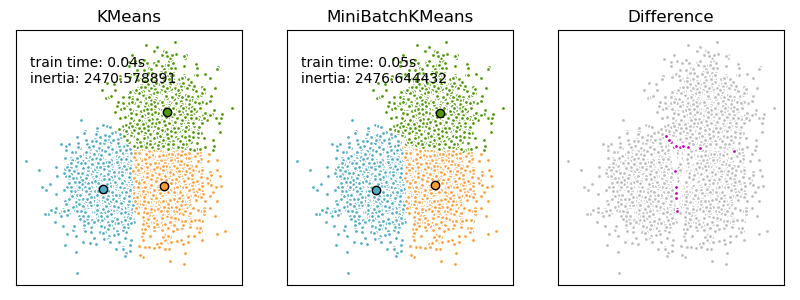n_clusters 这个就是k值，设置的簇的个数
init 簇心初始化的方法，有k-means++初始化方法，随机（random）初始化和自己指定样本（an ndarray），默认为k-means++，一般用默认的就可以，k-means++初始化效果也比较好
max_iter 最大迭代次数，这个参数主要是为了防止k-means不收敛，到达最大迭代次数后算法就终止
batch_size mini batch的样本个数，这个如果数据集很大，样本很多，可以设置的大些。
verbose 详细模式，就是设置为true的话会打出运行时的细节过程，建议设置为true，因为这样能更好的知道程序运行到什么程度了。
compute_labels 当聚类完成后，计算每个样本指派的簇心label和计算整个数据集的稳定性（inertia），默认为true，建议用true。
tol 容忍的最小误差，当误差小于tol时算法就会终止
max_no_improvement 用于早停（early stopping）的，即连续多少个Mini Batch没有改善聚类效果的话，算法就停止了。默认为为10，用默认值就好了。
init_size 用于簇心初始值候选的样本个数，为了加速簇心的初始化，相当于先从全部样本里随机挑init_size大小的样本作为候选集，簇心初始化从这个候选集里选。默认是batch_size的3倍，用默认值就好，我们只要设置batch_size就可以了。**有个注意点就是init_size的值要大于n_clusters的值。**我一般n_clusters设置2000的话，batch_size会设置1500-2000左右。
n_init 用不同的初始化簇心运行算法的次数。这里和KMeans里的n_init有一点不一样，KMeans类里的n_init是用同样的训练集数据来跑不同的初始化质心，然后选一个最好的结果。而MiniBatchKMeans类的n_init则是每次跑一个初始化簇心时就随机采样出一部分数据，在这个小数据集上跑，比如如果我设置n_init为3，则跑第一个簇心时随机采样一部分数据看看效果，跑第二个簇心时随机采样一部分数据看看效果，以此类推。然后挑出最好的初始化簇心来在整个数据集上跑，因此整个数据集上就跑一次。默认值为3，用默认值就可以。（关于这个参数，大家去看下源码和运行时设置verbose=True打出来的详细运行情况就一目了然了。）

from sklearn.cluster import MiniBatchKMeans
mini_batch_y_pred = MiniBatchKMeans(n_clusters=10, batch_size=50, verbose = 1, random_state=1).fit_predict(X)
plt.scatter(X[:,0], X[:,1],c = mini_batch_y_pred, marker = 'o', cmap = plt.cm.get_cmap("jet",10))
plt.xlabel('k = 10', fontsize=15)
plt.show()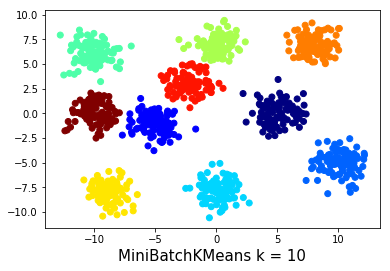: 刘建平Pinard博客《用scikit-learn学习K-Means聚类》
: 范淼等《python机器学习及实践》